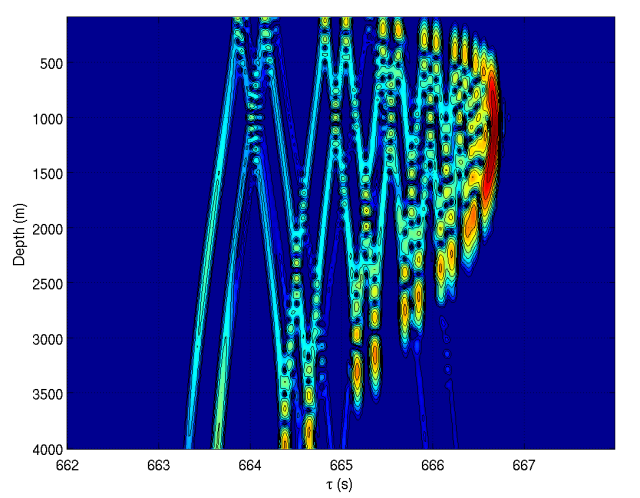# Calculating Acoustic Modes in Matlab

This is a page to download code for calculating acoustic modes used for simulating acoustic propagation over long ranges in deep water. Other hints for using this code and benchmarks can also be found here.

## Codes for Acoustic Propagation

• RAM in Fortran 95. A package for calculating acoustic propagation using the RAM Parabolic Equation approach and Fortran 95.
• RAM in Matlab. A package for calculating acoustic propagation using the RAM Parabolic Equation approach and matlab.
• Eigenray A package for calculating acoustic rays and travel times by ray tracing.

## Motivation

Regarding acoustic modes, there were two issues that nagged at me for years. The first is that it always seemed to me that solving the second order differential equation for modes (either acoustic or internal wave) should be quite easy in Matlab as an eigenvalue problem. The second is that no simple code was readily available to find the acoustic modes. (One readily-available suite of matlab scripts solves for the modes using Chebyshev polynomials; it seems to work quite well as measured by both speed and accuracy.) In any case, after a weekend of fussing around with the problem and consulting the Computational Ocean Acoustics book of Jensen et al., I developed this code below. In the end it was rather simple, but the devil is in the details, of course.

The code for calculating modes using Chebyshev polynomials (due to M. Dzieciuch) can be downloaded from the Ocean Acoustics Library: http://oalib.hlsresearch.com/The amodes.m script is stand alone. It is designed to be a drop in replacement for chebmode.m.
• amodes.m: Matlab script for calculating modes.
• modetest.m: Matlab example driver script; compares resulting modes to modes by Chebyshev polynomials.
• cssprofile.m: Matlab script for the canonical sound speed profile (from M. Dzieciuch) used in modetest.m.

## Computational Approach

The integration technique implemented to solve the second order differential equation for the modes is Numerov's Method, following the hint in Jensen et al. Computational Ocean Acoustics.

The method is implemented by discretizing the differential equation and setting up an eigenvalue problem. The matlab routine "eigs.m" is then used to solve for the desired number of eigenfunctions/eigenvalues.

While I amused myself in implementing this code, I have found it to be rather slow compared to the Chebyshev polynomial approach. The main problem is that the sound speed profile has to be discretized to at least 1 to 5 m, so that the eigenvalues/eigenfunctions have to be solved using a 5000X5000 to 1000X1000 sized matrix for a depth of 5000 m. Still, its not too bad, and a grid size of 5-m seems to be sufficient to reproduce the Chebyshev polynomal modes, while taking just a little longer (1.5 times as long?).

So at the moment, it is just an educational code.

It may be that this code can be more readily adapted to include the effects of the sea floor. Certainly, it can likely be adapted to solve for internal wave modes, using buoyancy rather than sound speed.

I am still a little puzzled as to how to implement the boundary conditions with this approach. I will have to work on sorting that out, particularly for internal wave modes which have a non-zero surface boundary condition.Simple range independent test case using the canonical sound speed profile at 1000 km. The modes were calculated with amode.m, while the calculation of the acoustic field was done using the rimodeex.m script of the Chebyshev polynomial package. A sound speed profile grid spacing of 10-m was used for this calculation. 10 m is rather pushing it; 5 m or less is noticeably more accurate.

-- Brian Dushaw - 09 Feb 2009

Copyright © 2008-2015 by the contributing authors. All material on this collaboration platform is the property of the contributing authors.